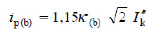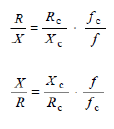• Sales
• Library Model Request
• Technical Support

# IEC 909 Loop/Meshed Definition

A meshed network bus is defined as:
1. There is at least a loop in the project.
2. Buses connected to the source through buses in a loop, and R/X is bigger than 0.3.

Loop/Mesh Definiteion:
1. To the faulted bus, if any fault current contribution from a source will reach faulted bus through a loop, then the network is considered meshed for the faulted bus. Otherwise it is considered non-meshed.

From IEC standard:
4.3.1.2 Short circuits in meshed networks

When calculating the peak short-circuit current ip in meshed networks, equation (54) shall be used with κ determined using one of the following methods a), b), or c).

a) Uniform ratio R/X or X/R

For this method the factor κ is determined from figure 15 taking the smallest ratio of R/X or the largest ratio of X/R of all branches of the network.

It is only necessary to choose the branches which carry partial short-circuit currents at the nominal voltage corresponding to the short-circuit location and branches with transformers adjacent to the short-circuit location. Any branch may be a series combination of several impedances.

b) Ratio R/X or X/R at the short-circuit location

For this method the factor κ is multiplied by a factor 1,15 to cover inaccuracies caused by using the ratio Rk / Xk from a network reduction with complex impedances.As long as R/X remains smaller than 0,3 in all branches, it is not necessary to use the factor 1,15. It is not necessary for the product 1,15 ⋅ κ(b) to exceed 1,8 in low-voltage networks or to exceed 2,0 in medium- and high-voltage networks.

The factor κ(b) is found from figure 15 for the ratio Rk/Xk given by the short-circuit impedance Zk = Rk + jXk at the short-circuit location F, calculated for frequency f = 50 Hz or 60 Hz.

c) Equivalent frequency fc

An equivalent impedance Zc of the system as seen from the short-circuit location is calculated assuming a frequency fc = 20 Hz (for a nominal frequency of f = 50 Hz) or fc = 24 Hz (for a nominal frequency of f = 60 Hz). The R/X or X/R ratio is then determined according to equation (59). where
Zc = Rc + jXc is the equivalent impedance of the system as seen from the short-circuit location
for the assumed frequency fc;
Rc is the real part of Zc (Rc is generally not equal to the R at nominal frequency)
Xc is the imaginary part of Zc (Xc is generally not equal to the X at nominal frequency).

The factor κ is found from figure 15 using the R/X or X/R ratio from equation (59), or with equation (55). Method c) is recommended in meshed networks (see IEC 60909-1).

When using this method in meshed networks with transformers, generators and power station units, the impedance correction factors KT, KG and KS, respectively KSO, shall be introduced with the same values as for the 50 Hz or 60 Hz calculations.

New mesh rules:
The situation of two sources going to same branches then to the bus is also considered a mesh network.